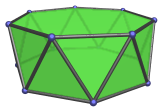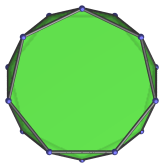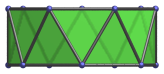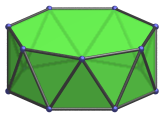# The Heptagonal Antiprism

The heptagonal antiprism is a 3D uniform polyhedron bounded by 16 polygons (2 heptagons and 14 triangles), 28 edges, and 14 vertices.## Projections

The following are some commonly-encountered views of the heptagonal antiprism:

Projection DescriptionHeptagon-centered parallel projection.Parallel to a pair of opposite lateral edges.Parallel to a pair of opposite triangles.Vertex-centered parallel projection.

## Coordinates

The Cartesian coordinates of the heptagonal antiprism, centered on the origin and having edge length 2, are:

• (0, r, H)
• (±A, B, H)
• (±C, −D, H)
• (±1, −h, H)
• (0, −r, −H)
• (±A, −B, −H)
• (±C, D, −H)
• (±1, h, −H)

where r, A, B, C, D, h, and H are roots of the following polynomials within the indicated ranges:

 7r6 − 56r4 + 112r2 − 64 = 0, 2≤r≤3 A3 − A2 − 2A + 1 = 0, 1≤A≤2 7B6 − 21B4 + 14B2 − 1 = 0, 1≤B≤2 C3 − 2C2 − C + 1 = 0, 2≤C≤3 7D6 − 14D4 + 7D2 − 1 = 0, 0≤D≤1 7h6 − 35h4 + 21h2 − 1 = 0, 2≤h≤3 7H6 − 7H4 + 1 = 0, 0.8≤H≤0.9

r and h are the out-radius and in-radius, respectively, of a regular heptagon of edge length 2. H is half the height of the antiprism.

Their approximate values are:

• r = 2.304764870962486
• A = 1.801937735804838
• B = 1.436997392727370
• C = 2.246979603717467
• D = 0.512858431636277
• h = 2.076521396572336
• H = 0.858473196494555

Last updated 24 Jul 2020.